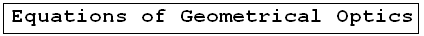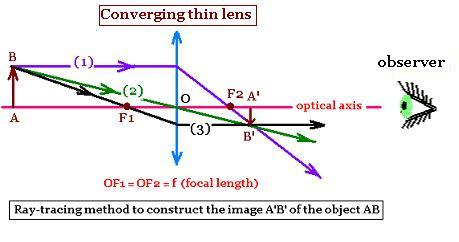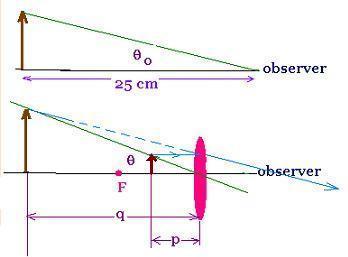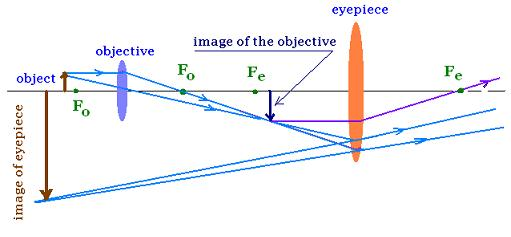ÂGeometrical Optics: Geometrical Optics & Physics Optics. a Huygens Principle: Wavefronts. a Reflection: The first law of Geometrical Optics. a Refraction: The second law of Geometrical Optics: Snell's law. a Critical angle: Internal total reflection. a images formed by reflection Spherical concave mirrors. Mirror equation. a images formed by refraction Spherical convex mirrors .. a Fermat's principle: Fermat's principle for reflection and refraction.. a lenses: Thin lenses and Lens-makers' equation .. a prism: Prism, colors minimum deviation .. a Human eye: near point, far point, nearsightedness, farsightedness.. a Microscope: Microscope: simple, compound, and magnification.. a Telescope: Telescope: magnification.. a Resolution: optical microscope, electron microscope .. a dispersion: dispersion, more about the refractive index, and colors of a prism .. a parallel sheet: deviation by a parallel sheet .. a thin films : thin films and Newton's rings.. a Newton's rings: thin films and Newton's rings.. a Descartes rainbow: Primary and secondary rainbows .. a Some applications More fun with Optics .. a Optics calculator: Optics calculator: all the related calculations .. a home The fundamental, and just this .. a scientificSentence a __________________ a

Geometrical Optics: Applications: compound microscope
Optics - Â  Â  Geometrical Optics..

## Simple and compound microscopeWe want to form the image of an object seen through a converging lens. If the object is placed at left infinity, its image will be located exactly at the right focal point. If the object is placed at the left of the focal point of this converging lens, the related image is real and inverted. The more we approch to this point from the left, the more the related image is large, and more far from the lens. If it is placed exactly at the focal point, the constructing rays of the image are parallel and the image is at the infinity.Between the focal point and the center of the lens, the image is virtual, upright, and enlarged comming from the left. There is absolutely no image between the center of a converging lens and its right focal point.

### 1. The simple magnifierThe more an object is near the observer, the more the related angle formed by that object is large. For the humain eye, the related length is about 25 cm. We write tg Î¸o = h/25 cm. The length "h" is the height of the object.
tg Î¸ = h/p
The angular magnification is defined as

m = Î¸/Î¸o = (h/p)/(h/25 cm) = 25cm/p

The angular magnification is maximum when the angle Î¸ is maximum, that is when the image formed by the converging lens is at q = - 25 cm.
Now, We want an expression of the angular magnification "m".
1/p + 1/q = 1/f, so
1/p = 1/f - 1/q = (q - f)/qf, then:
p = qf/(q - f)

The angles Î¸ and Î¸o are small, so Î¸o = tg Î¸o = h/25 cm, and Î¸ = tg Î¸ = h/p
Therefore:
mmax = (h/p)/(h/25cm) = 25cm/P = 25cm(q - f)/qf = 25cm(-25 cm - f)/(-25 cm)f with q = - 25 cm, we have:
mmax = 25 cm(25 cm - f)/25 cm f = 25 cm /f + 1

Exactly, when the object is near the focal point, (p = f) , the image should be formed at infinity to minimize eyestrain. we have:

m = (h/f)/(h/25 cm)= 25cm/f

This is the magnification of the simple magnifier.

### 2. Compound microscopeA compound microscope gives greater magnification than a single lens. It contains two converging lenses: The objective lens that has a short focal length fo (< 1 cm) , and the eyepiece that has a focal length, fe of a few cm.

The linear magnification by the objective is mo = - p/q
The angular magnification by the eyepiece of the microscope is me = 25 cm / e The total magnification mt of the microscope is the product of the magnification by the objective = mo = - q/p, and the magnification by the eyepiece = me = 25cm/fe

mt = - (q/p)(25cm/fe)

Exactly, when the object is near the focal point, (p = fo), and the first image is located at focal point of the eyepiece, the second image virtual, inverted and magnified is formed at infinity.
If we set:
T = distance between the two focal points fe and fe. It is called the tube length T. It is often set to 160 mm, then:
mo = - q/p = - T/fo
therefore:

mt = - (T/f0)(25cm/fe) = - 25cm T/f0fe)

This is the often used magnification of the compound microscope.

Â

 @import url(http://www.google.com/cse/api/branding.css);Custom Search Â© 2009. The scientificsentence . All rights reserved.